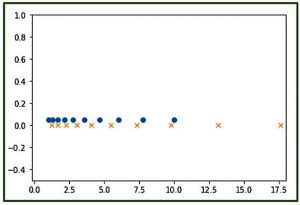# numpy.logspace() in Python

The numpy.logspace() function returns number spaces evenly w.r.t interval on a log scale.
Syntax :

```numpy.logspace(start,
stop,
num = 50,
endpoint = True,
base = 10.0,
dtype = None)```

Parameters :

```-> start    : [float] start(base ** start) of interval range.
-> stop     : [float] end(base ** stop) of interval range
-> endpoint : [boolean, optional]If True, stop is the last sample. By default, True
-> num      : [int, optional] No. of samples to generate
-> base     : [float, optional] Base of log scale. By default, equals 10.0
-> dtype    : type of output array
```

Return :

```-> ndarray
```

Code 1 : Explaining the use of logspace()

 `# Python Programming illustrating ` `# numpy.logspace method ` ` `  `import` `numpy as geek ` ` `  `# base = 11 ` `print``(``"B\n"``, geek.logspace(``2.0``, ``3.0``, num``=``5``, base ``=` `11``)) ` ` `  `# base = 10 ` `print``(``"B\n"``, geek.logspace(``2.0``, ``3.0``, num``=``5``)) ` ` `  `# base = 10, dtype = int ` `print``(``"B\n"``, geek.logspace(``2.0``, ``3.0``, num``=``5``, dtype ``=` `int``)) `

Output :

```B
[  121.           220.36039471   401.31159963   730.8527479   1331.        ]
B
[  100.           177.827941     316.22776602   562.34132519  1000.        ]
B
[ 100  177  316  562 1000]
```

Code 2 : Graphical Representation of numpy.logspace() using matplotlib module – pylab

 `# Graphical Represenation of numpy.logspace() ` `import` `numpy as geek ` `import` `pylab as p ` ` `  `# Start = 0 ` `# End = 2 ` `# Samples to generate = 10 ` `x1 ``=` `geek.logspace(``0``, ``1``, ``10``) ` `y1 ``=` `geek.zeros(``10``) ` ` `  `# Start = 0.1 ` `# End = 1.5 ` `# Samples to generate = 12 ` `x2 ``=` `geek.logspace(``0.1``, ``1.5``, ``12``) ` `y2 ``=` `geek.zeros(``12``) ` ` `  `p.plot(x1, y1``+``0.05``, ``'o'``) ` `p.xlim(``-``0.2``, ``18``) ` `p.ylim(``-``0.5``, ``1``) ` `p.plot(x2, y2, ``'x'``) `

Output :Note :
These NumPy-Python programs won’t run on onlineID, so run them on your systems to explore them

Similiar methods :

• arange
• linspace
• This article is contributed by Mohit Gupta_OMG 😀. If you like GeeksforGeeks and would like to contribute, you can also write an article using contribute.geeksforgeeks.org or mail your article to contribute@geeksforgeeks.org. See your article appearing on the GeeksforGeeks main page and help other Geeks.

Attention geek! Strengthen your foundations with the Python Programming Foundation Course and learn the basics.

To begin with, your interview preparations Enhance your Data Structures concepts with the Python DS Course.

My Personal Notes arrow_drop_up
Article Tags :

Be the First to upvote.

Please write to us at contribute@geeksforgeeks.org to report any issue with the above content.